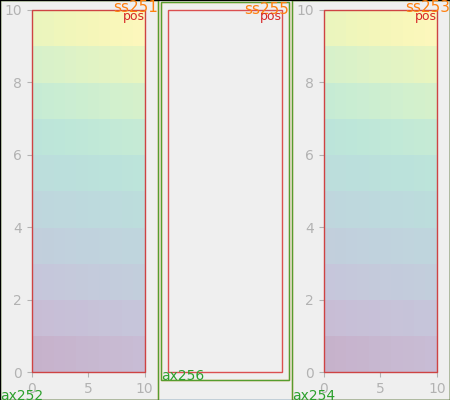# Constrained Layout Guide¶

How to use constrained-layout to fit plots within your figure cleanly.

constrained_layout automatically adjusts subplots and decorations like legends and colorbars so that they fit in the figure window while still preserving, as best they can, the logical layout requested by the user.

constrained_layout is similar to tight_layout, but uses a constraint solver to determine the size of axes that allows them to fit.

Warning

As of Matplotlib 2.2, Constrained Layout is experimental. The behaviour and API are subject to change, or the whole functionality may be removed without a deprecation period. If you require your plots to be absolutely reproducible, get the Axes positions after running Constrained Layout and use `ax.set_position()` in your code with `constrained_layout=False`.

## Simple Example¶

In Matplotlib, the location of axes (including subplots) are specified in normalized figure coordinates. It can happen that your axis labels or titles (or sometimes even ticklabels) go outside the figure area, and are thus clipped.

```# sphinx_gallery_thumbnail_number = 18

#import matplotlib
#matplotlib.use('Qt5Agg')

import warnings

import matplotlib.pyplot as plt
import numpy as np
import matplotlib.colors as mcolors
import matplotlib.gridspec as gridspec

import matplotlib._layoutbox as layoutbox

plt.rcParams['savefig.facecolor'] = "0.8"
plt.rcParams['figure.figsize'] = 4.5, 4.

def example_plot(ax, fontsize=12, nodec=False):
ax.plot([1, 2])

ax.locator_params(nbins=3)
if not nodec:
ax.set_xlabel('x-label', fontsize=fontsize)
ax.set_ylabel('y-label', fontsize=fontsize)
ax.set_title('Title', fontsize=fontsize)
else:
ax.set_xticklabels('')
ax.set_yticklabels('')

fig, ax = plt.subplots()
example_plot(ax, fontsize=24)
```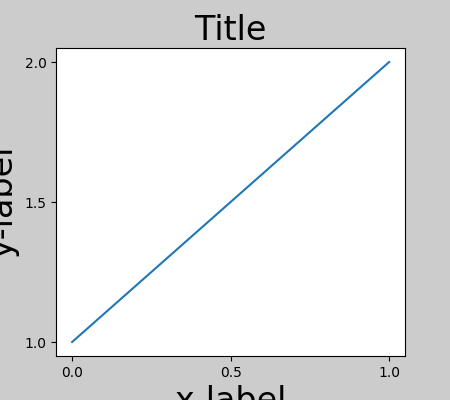To prevent this, the location of axes needs to be adjusted. For subplots, this can be done by adjusting the subplot params (Move the edge of an axes to make room for tick labels). However, specifying your figure with the `constrained_layout=True` kwarg will do the adjusting automatically.

```fig, ax = plt.subplots(constrained_layout=True)
example_plot(ax, fontsize=24)
```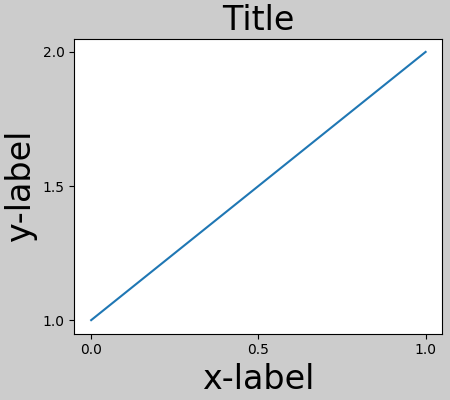When you have multiple subplots, often you see labels of different axes overlapping each other.

```fig, axs = plt.subplots(2, 2, constrained_layout=False)
for ax in axs.flatten():
example_plot(ax)
```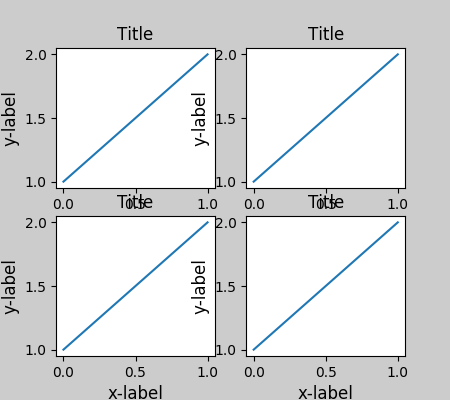Specifying `constrained_layout=True` in the call to `plt.subplots` causes the layout to be properly constrained.

```fig, axs = plt.subplots(2, 2, constrained_layout=True)
for ax in axs.flatten():
example_plot(ax)
```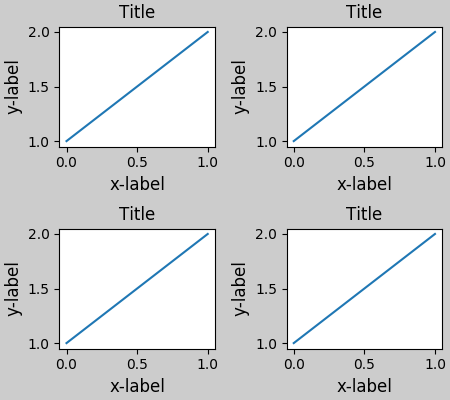## Colorbars¶

If you create a colorbar with the `colorbar()` command you need to make room for it. `constrained_layout` does this automatically. Note that if you specify `use_gridspec=True` it will be ignored because this option is made for improving the layout via `tight_layout`.

Note

For the `pcolormesh` kwargs (`pc_kwargs`) we use a dictionary. Below we will assign one colorbar to a number of axes each containing a `ScalarMappable`; specifying the norm and colormap ensures the colorbar is accurate for all the axes.

```arr = np.arange(100).reshape((10, 10))
norm = mcolors.Normalize(vmin=0., vmax=100.)
# see note above: this makes all pcolormesh calls consistent:
pc_kwargs = {'rasterized':True, 'cmap':'viridis', 'norm':norm}
fig, ax = plt.subplots(figsize=(4, 4), constrained_layout=True)
im = ax.pcolormesh(arr, **pc_kwargs)
fig.colorbar(im, ax=ax, shrink=0.6)
```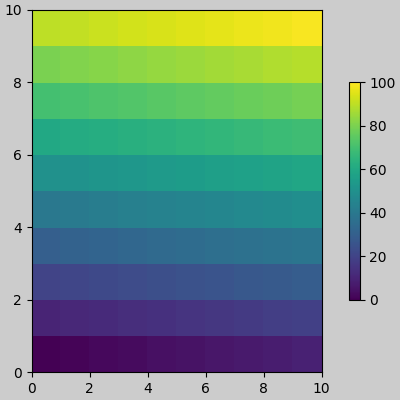If you specify a list of axes (or other iterable container) to the `ax` argument of `colorbar`, constrained_layout will take space from all # axes that share the same gridspec.

```fig, axs = plt.subplots(2, 2, figsize=(4, 4), constrained_layout=True)
for ax in axs.flatten():
im = ax.pcolormesh(arr, **pc_kwargs)
fig.colorbar(im, ax=axs, shrink=0.6)
```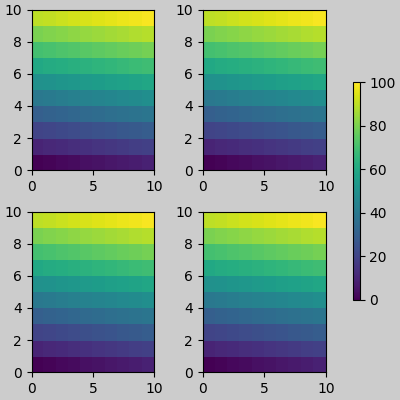Note that there is a bit of a subtlety when specifying a single axes as the parent. In the following, it might be desirable and expected for the colorbars to line up, but they don’t because the colorbar paired with the bottom axes is tied to the subplotspec of the axes, and hence shrinks when the gridspec-level colorbar is added.

```fig, axs = plt.subplots(3, 1, figsize=(4, 4), constrained_layout=True)
for ax in axs[:2]:
im = ax.pcolormesh(arr, **pc_kwargs)
fig.colorbar(im, ax=axs[:2], shrink=0.6)
im = axs.pcolormesh(arr, **pc_kwargs)
fig.colorbar(im, ax=axs, shrink=0.6)
```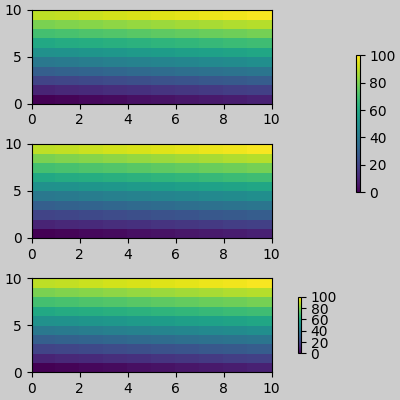The API to make a single-axes behave like a list of axes is to specify it as a list (or other iterable container), as below:

```fig, axs = plt.subplots(3, 1, figsize=(4, 4), constrained_layout=True)
for ax in axs[:2]:
im = ax.pcolormesh(arr, **pc_kwargs)
fig.colorbar(im, ax=axs[:2], shrink=0.6)
im = axs.pcolormesh(arr, **pc_kwargs)
fig.colorbar(im, ax=[axs], shrink=0.6)
```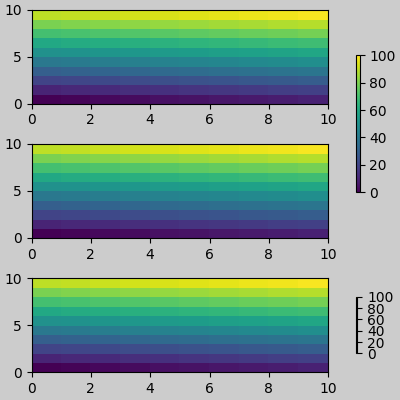## Suptitle¶

`constrained_layout` can also make room for `suptitle`.

```fig, axs = plt.subplots(2, 2, figsize=(4, 4), constrained_layout=True)
for ax in axs.flatten():
im = ax.pcolormesh(arr, **pc_kwargs)
fig.colorbar(im, ax=axs, shrink=0.6)
fig.suptitle('Big Suptitle')
```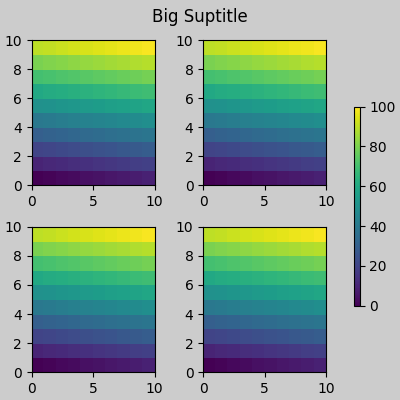## Legends¶

Legends can be placed outside of their parent axis. Constrained-layout is designed to handle this. However, constrained-layout does not handle legends being created via `fig.legend()` (yet).

```fig, ax = plt.subplots(constrained_layout=True)
ax.plot(np.arange(10), label='This is a plot')
ax.legend(loc='center left', bbox_to_anchor=(0.9, 0.5))
```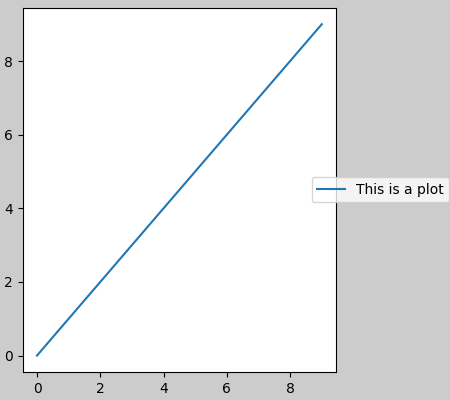However, this will steal space from a subplot layout:

```fig, axs = plt.subplots(2, 2, constrained_layout=True)
for ax in axs.flatten()[:-1]:
ax.plot(np.arange(10))
axs[1, 1].plot(np.arange(10), label='This is a plot')
axs[1, 1].legend(loc='center left', bbox_to_anchor=(0.9, 0.5))
```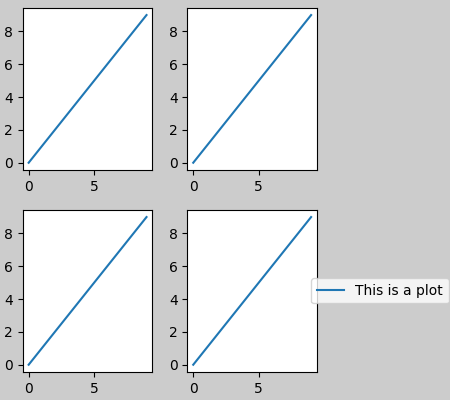For constrained_layout, we have implemented a padding around the edge of each axes. This padding sets the distance from the edge of the plot, and the minimum distance between adjacent plots. It is specified in inches by the keyword arguments `w_pad` and `h_pad` to the function `fig.set_constrained_layout_pads`:

```fig, axs = plt.subplots(2, 2, constrained_layout=True)
for ax in axs.flatten():
example_plot(ax, nodec=True)
ax.set_xticklabels('')
ax.set_yticklabels('')
hspace=0., wspace=0.)

fig, axs = plt.subplots(2, 2, constrained_layout=True)
for ax in axs.flatten():
example_plot(ax, nodec=True)
ax.set_xticklabels('')
ax.set_yticklabels('')
hspace=0., wspace=0.)
```
•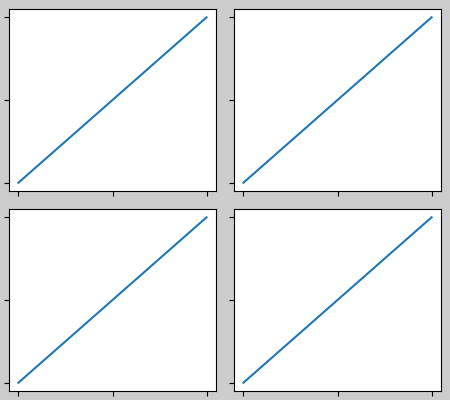•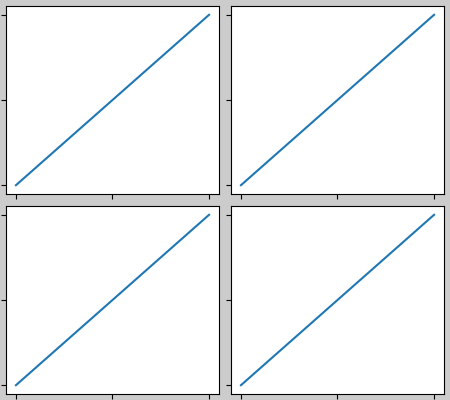Spacing between subplots is set by `wspace` and `hspace`. There are specified as a fraction of the size of the subplot group as a whole. If the size of the figure is changed, then these spaces change in proportion. Note in the blow how the space at the edges doesn’t change from the above, but the space between subplots does.

```fig, axs = plt.subplots(2, 2, constrained_layout=True)
for ax in axs.flatten():
example_plot(ax, nodec=True)
ax.set_xticklabels('')
ax.set_yticklabels('')
hspace=0.2, wspace=0.2)
```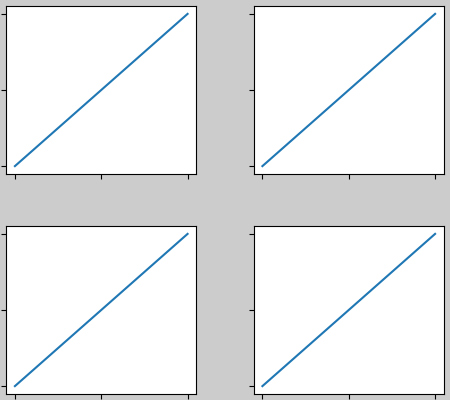### Spacing with colorbars¶

Colorbars still respect the `w_pad` and `h_pad` values. However they will be `wspace` and `hsapce` apart from other subplots. Note the use of a `pad` kwarg here in the `colorbar` call. It defaults to 0.02 of the size of the axis it is attached to.

```fig, axs = plt.subplots(2, 2, constrained_layout=True)
for ax in axs.flatten():
pc = ax.pcolormesh(arr, **pc_kwargs)
ax.set_xticklabels('')
ax.set_yticklabels('')
hspace=0.2, wspace=0.2)
```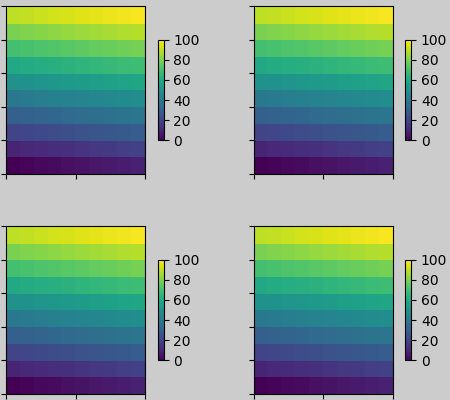In the above example, the colorbar will not ever be closer than 2 pts to the plot, but if we want it a bit further away, we can specify its value for `pad` to be non-zero.

```fig, axs = plt.subplots(2, 2, constrained_layout=True)
for ax in axs.flatten():
pc = ax.pcolormesh(arr, **pc_kwargs)
ax.set_xticklabels('')
ax.set_yticklabels('')
hspace=0.2, wspace=0.2)
```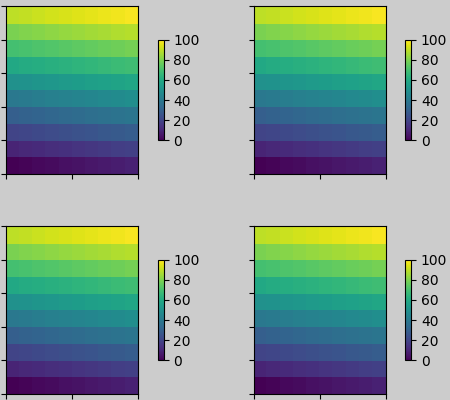### rcParams¶

There are five `rcParams` that can be set, either in a script or in the `matplotlibrc` file. They all have the prefix `figure.constrained_layout`:

• `use`: Whether to use constrained_layout. Default is False
• `w_pad`, `h_pad` Padding around axes objects.
Float representing inches. Default is 3./72. inches (3 pts)
• `wspace`, `hspace` Space between subplot groups.
Float representing a fraction of the subplot widths being separated. Default is 0.02.
```plt.rcParams['figure.constrained_layout.use'] = True
fig, axs = plt.subplots(2, 2, figsize=(3, 3))
for ax in axs.flatten():
example_plot(ax)
```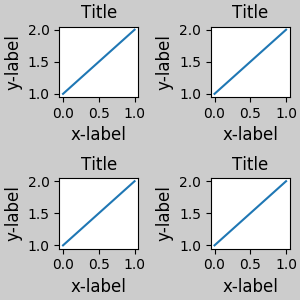## Use with GridSpec¶

constrained_layout is meant to be used with `subplots()` or `GridSpec()` and `add_subplot()`.

```fig = plt.figure(constrained_layout=True)

gs1 = gridspec.GridSpec(2, 1, figure=fig)

example_plot(ax1)
example_plot(ax2)
```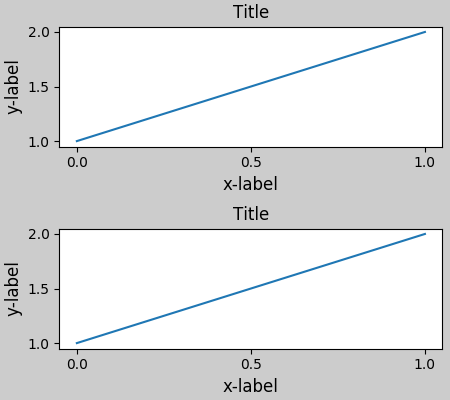More complicated gridspec layouts are possible…

```fig = plt.figure(constrained_layout=True)

gs0 = gridspec.GridSpec(1, 2, figure=fig)

gs1 = gridspec.GridSpecFromSubplotSpec(2, 1, gs0)

example_plot(ax1)
example_plot(ax2)

gs2 = gridspec.GridSpecFromSubplotSpec(3, 1, gs0)

for ss in gs2:
example_plot(ax)
ax.set_title("")
ax.set_xlabel("")

ax.set_xlabel("x-label", fontsize=12)
```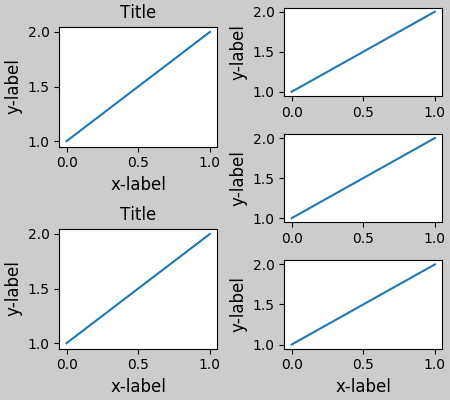Note that in the above the left and columns don’t have the same vertical extent. If we want the top and bottom of the two grids to line up then they need to be in the same gridspec:

```fig = plt.figure(constrained_layout=True)

gs0 = gridspec.GridSpec(6, 2, figure=fig)

example_plot(ax1)
example_plot(ax2)

example_plot(ax)
example_plot(ax)
example_plot(ax)
```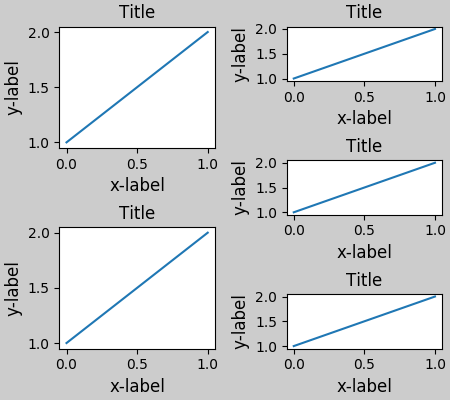This example uses two gridspecs to have the colorbar only pertain to one set of pcolors. Note how the left column is wider than the two right-hand columns because of this. Of course, if you wanted the subplots to be the same size you only needed one gridspec.

```def docomplicated(suptitle=None):
fig = plt.figure(constrained_layout=True)
gs0 = gridspec.GridSpec(1, 2, figure=fig, width_ratios=[1., 2.])
gsl = gridspec.GridSpecFromSubplotSpec(2, 1, gs0)
gsr = gridspec.GridSpecFromSubplotSpec(2, 2, gs0)

for gs in gsl:
example_plot(ax)
axs = []
for gs in gsr:
pcm = ax.pcolormesh(arr, **pc_kwargs)
ax.set_xlabel('x-label')
ax.set_ylabel('y-label')
ax.set_title('title')

axs += [ax]
fig.colorbar(pcm, ax=axs)
if suptitle is not None:
fig.suptitle(suptitle)

docomplicated()
```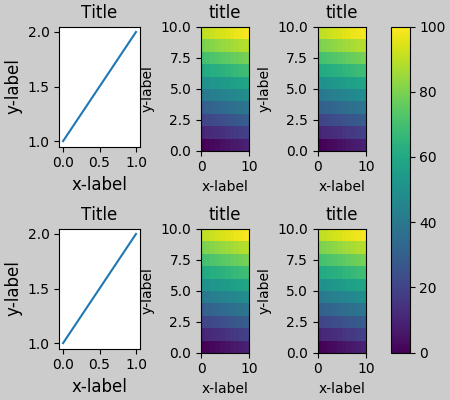## Manually setting axes positions¶

There can be good reasons to manually set an axes position. A manual call to `ax.set_position()` will set the axes so constrained_layout has no effect on it anymore. (Note that constrained_layout still leaves the space for the axes that is moved).

```fig, axs = plt.subplots(1, 2, constrained_layout=True)
example_plot(axs, fontsize=12)
axs.set_position([0.2, 0.2, 0.4, 0.4])
```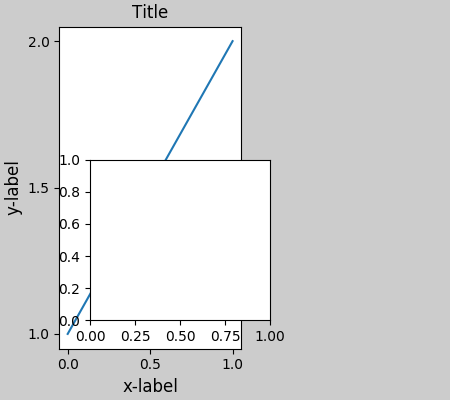If you want an inset axes in data-space, you need to manually execute the layout using `fig.execute_constrained_layout()` call. The inset figure will then be properly positioned. However, it will not be properly positioned if the size of the figure is subsequently changed. Similarly, if the figure is printed to another backend, there may be slight changes of location due to small differences in how the backends render fonts.

```from matplotlib.transforms import Bbox

fig, axs = plt.subplots(1, 2, constrained_layout=True)
example_plot(axs, fontsize=12)
fig.execute_constrained_layout()
# put into data-space:
bb_data_ax2 = Bbox.from_bounds(0.5, 1., 0.2, 0.4)
disp_coords = axs.transData.transform(bb_data_ax2)
fig_coords_ax2 = fig.transFigure.inverted().transform(disp_coords)
bb_ax2 = Bbox(fig_coords_ax2)
```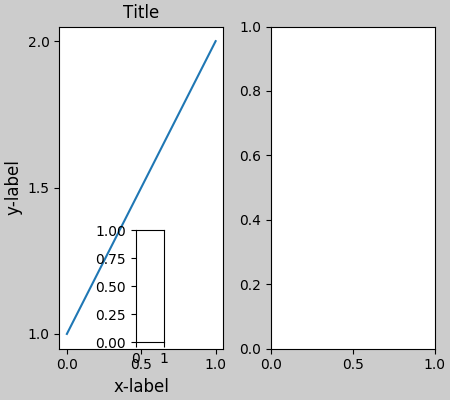## Limitations¶

### Incompatible functions¶

`constrained_layout` will not work on subplots created via the `subplot` command. The reason is that each of these commands creates a separate `GridSpec` instance and `constrained_layout` uses (nested) gridspecs to carry out the layout. So the following fails to yield a nice layout:

```fig = plt.figure(constrained_layout=True)

ax1 = plt.subplot(221)
ax2 = plt.subplot(223)
ax3 = plt.subplot(122)

example_plot(ax1)
example_plot(ax2)
example_plot(ax3)
```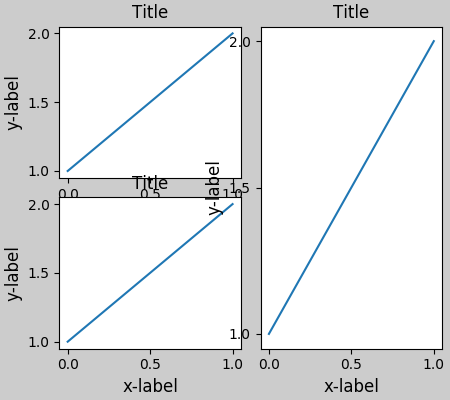Of course that layout is possible using a gridspec:

```fig = plt.figure(constrained_layout=True)
gs = gridspec.GridSpec(2, 2, figure=fig)

example_plot(ax1)
example_plot(ax2)
example_plot(ax3)
```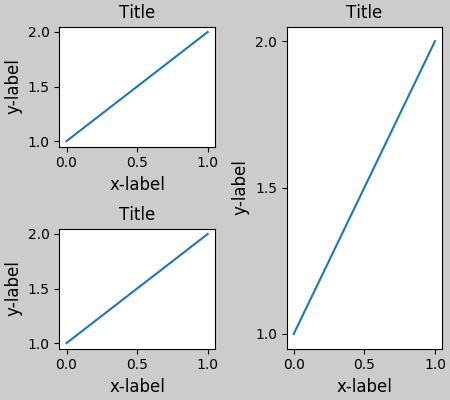Similarly, `subplot2grid()` doesn’t work for the same reason: each call creates a different parent gridspec.

```fig = plt.figure(constrained_layout=True)

ax1 = plt.subplot2grid((3, 3), (0, 0))
ax2 = plt.subplot2grid((3, 3), (0, 1), colspan=2)
ax3 = plt.subplot2grid((3, 3), (1, 0), colspan=2, rowspan=2)
ax4 = plt.subplot2grid((3, 3), (1, 2), rowspan=2)

example_plot(ax1)
example_plot(ax2)
example_plot(ax3)
example_plot(ax4)
```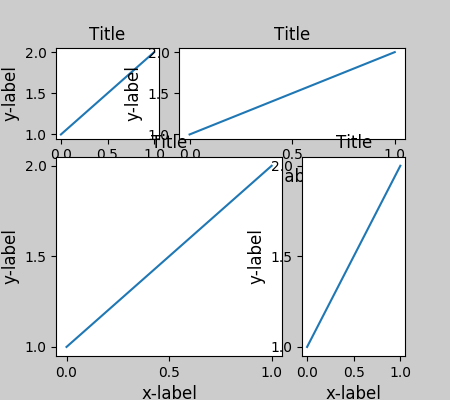The way to make this plot compatible with `constrained_layout` is again to use `gridspec` directly

```fig = plt.figure(constrained_layout=True)
gs = gridspec.GridSpec(3, 3, figure=fig)

example_plot(ax1)
example_plot(ax2)
example_plot(ax3)
example_plot(ax4)
```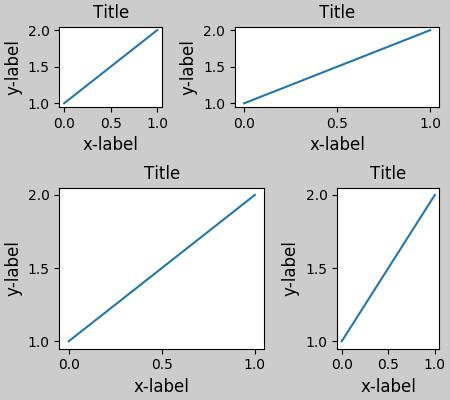### Other Caveats¶

• `constrained_layout` only considers ticklabels, axis labels, titles, and legends. Thus, other artists may be clipped and also may overlap.
• It assumes that the extra space needed for ticklabels, axis labels, and titles is independent of original location of axes. This is often true, but there are rare cases where it is not.
• There are small differences in how the backends handle rendering fonts, so the results will not be pixel-identical.

## Debugging¶

Constrained-layout can fail in somewhat unexpected ways. Because it uses a constraint solver the solver can find solutions that are mathematically correct, but that aren’t at all what the user wants. The usual failure mode is for all sizes to collapse to their smallest allowable value. If this happens, it is for one of two reasons:

1. There was not enough room for the elements you were requesting to draw.
2. There is a bug - in which case open an issue at https://github.com/matplotlib/matplotlib/issues.

If there is a bug, please report with a self-contained example that does not require outside data or dependencies (other than numpy).

## Notes on the algorithm¶

The algorithm for the constraint is relatively straightforward, but has some complexity due to the complex ways we can layout a figure.

### Figure layout¶

Figures are laid out in a hierarchy:

1. Figure: `fig = plt.figure()`
1. Gridspec `gs0 = gridspec.GridSpec(1, 2, figure=fig)`

1. Subplotspec: `ss = gs[0, 0]`
1. Axes: `ax0 = fig.add_subplot(ss)`
2. Subplotspec: `ss = gs[0, 1]`
1. Gridspec: `gsR = gridspec.GridSpecFromSubplotSpec(2, 1, ss)`

• Subplotspec: ss = gsR[0, 0]

• Axes: `axR0 = fig.add_subplot(ss)`
• Subplotspec: ss = gsR[1, 0]

• Axes: `axR1 = fig.add_subplot(ss)`

Each item has a layoutbox associated with it. The nesting of gridspecs created with `GridSpecFromSubplotSpec` can be arbitrarily deep.

Each `Axes` has two layoutboxes. The first one `ax._layoutbox` represents the outside of the Axes and all its decorations (i.e. ticklabels, axis labels, etc.). The second layoutbox corresponds to the Axes’ `ax.position`, which sets where in the figure the spines are placed.

Why so many stacked containers? Ideally, all that would be needed are the Axes layout boxes. For the Gridspec case, a container is needed if the Gridspec is nested via `GridSpecFromSubplotSpec`. At the top level, it is desirable for symmetry, but it also makes room for `suptitle`.

For the Subplotspec/Axes case, Axes often have colorbars or other annotations that need to be packaged inside the Subplotspec, hence the need for the outer layer.

### Simple case: one Axes¶

For a single Axes the layout is straight forward. The Figure and outer Gridspec layoutboxes coincide. The Subplotspec and Axes boxes also coincide because the Axes has no colorbar. Note the difference between the red `pos` box and the green `ax` box is set by the size of the decorations around the Axes.

In the code, this is accomplished by the entries in `do_constrained_layout` like:

```ax._poslayoutbox.edit_left_margin_min(-bbox.x0 + pos.x0 + w_padt)
```
```from matplotlib._layoutbox import plot_children

fig, ax = plt.subplots(constrained_layout=True)
example_plot(ax, fontsize=24)
plot_children(fig, fig._layoutbox, printit=False)
```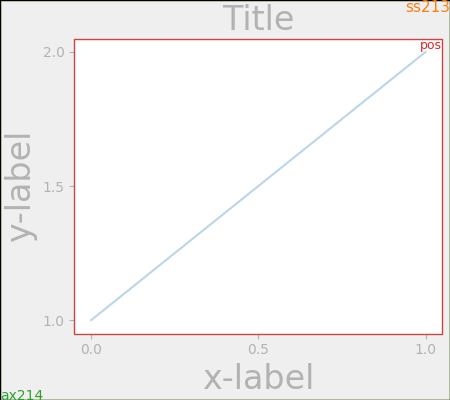### Simple case: two Axes¶

For this case, the Axes layoutboxes and the Subplotspec boxes still co-incide. However, because the decorations in the right-hand plot are so much smaller than the left-hand, so the right-hand layoutboxes are smaller.

The Subplotspec boxes are laid out in the code in the subroutine `arange_subplotspecs`, which simply checks the subplotspecs in the code against one another and stacks them appropriately.

The two `pos` axes are lined up. Because they have the same minimum row, they are lined up at the top. Because they have the same maximum row they are lined up at the bottom. In the code this is accomplished via the calls to `layoutbox.align`. If there was more than one row, then the same horizontal alignment would occur between the rows.

The two `pos` axes are given the same width because the subplotspecs occupy the same number of columns. This is accomplished in the code where `dcols0` is compared to `dcolsC`. If they are equal, then their widths are constrained to be equal.

While it is a bit subtle in this case, note that the division between the Subplotspecs is not centered, but has been moved to the right to make space for the larger labels on the left-hand plot.

```fig, ax = plt.subplots(1, 2, constrained_layout=True)
example_plot(ax, fontsize=32)
example_plot(ax, fontsize=8)
plot_children(fig, fig._layoutbox, printit=False)
```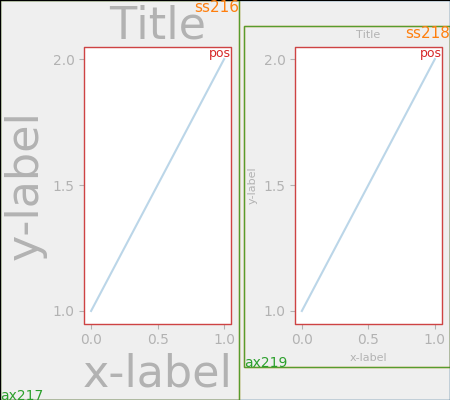### Two Axes and colorbar¶

Adding a colorbar makes it clear why the Subplotspec layoutboxes must be different from the axes layoutboxes. Here we see the left-hand subplotspec has more room to accommodate the `colorbar`, and that there are two green `ax` boxes inside the `ss` box.

Note that the width of the `pos` boxes is still the same because of the constraint on their widths because their subplotspecs occupy the same number of columns (one in this example).

The colorbar layout logic is contained in `make_axes` which call `_constrained_layout.layoutcolorbarsingle` for cbars attached to a single axes, and `_constrained_layout.layoutcolorbargridspec` if the colorbar is associated wiht a gridspec.

```fig, ax = plt.subplots(1, 2, constrained_layout=True)
im = ax.pcolormesh(arr, **pc_kwargs)
fig.colorbar(im, ax=ax, shrink=0.6)
im = ax.pcolormesh(arr, **pc_kwargs)
plot_children(fig, fig._layoutbox, printit=False)
```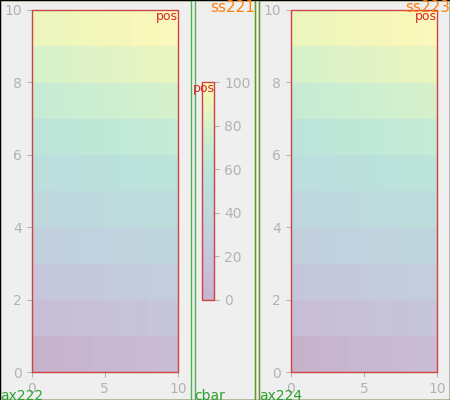### Colorbar associated with a Gridspec¶

This example shows the Subplotspec layoutboxes being made smaller by a colorbar layoutbox. The size of the colorbar layoutbox is set to be shrink smaller than the vertical extent of the `pos` layoutboxes in the gridspec, and it is made to be centered between those two points.

```fig, ax = plt.subplots(2, 2, constrained_layout=True)
for a in ax.flatten():
im = a.pcolormesh(arr, **pc_kwargs)
fig.colorbar(im, ax=ax, shrink=0.6)
plot_children(fig, fig._layoutbox, printit=False)
```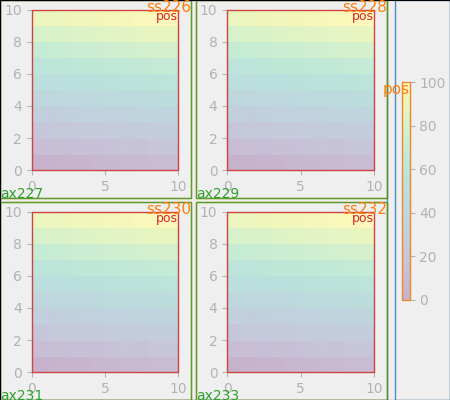### Uneven sized Axes¶

There are two ways to make axes have an uneven size in a Gridspec layout, either by specifying them to cross Gridspecs rows or columns, or by specifying width and height ratios.

The first method is used here. The constraint that makes the heights be correct is in the code where `drowsC < drows0` which in this case would be 1 is less than 2. So we constrain the height of the 1-row Axes to be less than half the height of the 2-row Axes.

Note

This algorithm can be wrong if the decorations attached to the smaller axes are very large, so there is an unaccounted-for edge case.

```fig = plt.figure(constrained_layout=True)
gs = gridspec.GridSpec(2, 2, figure=fig)
im = ax.pcolormesh(arr, **pc_kwargs)
im = ax.pcolormesh(arr, **pc_kwargs)
im = ax.pcolormesh(arr, **pc_kwargs)
plot_children(fig, fig._layoutbox, printit=False)
```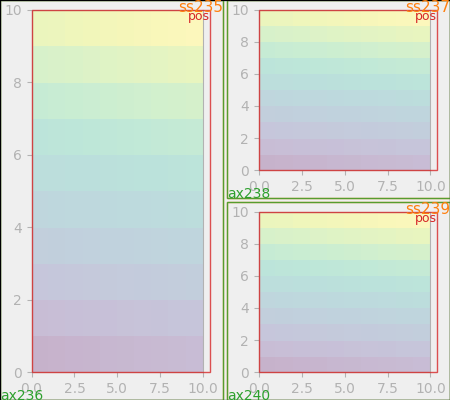Height and width ratios are accommodated with the same part of the code with the smaller axes always constrained to be less in size than the larger.

```fig = plt.figure(constrained_layout=True)
gs = gridspec.GridSpec(3, 2, figure=fig,
height_ratios=[1., 0.5, 1.5],
width_ratios=[1.2, 0.8])
im = ax.pcolormesh(arr, **pc_kwargs)
im = ax.pcolormesh(arr, **pc_kwargs)
im = ax.pcolormesh(arr, **pc_kwargs)
im = ax.pcolormesh(arr, **pc_kwargs)
plot_children(fig, fig._layoutbox, printit=False)
```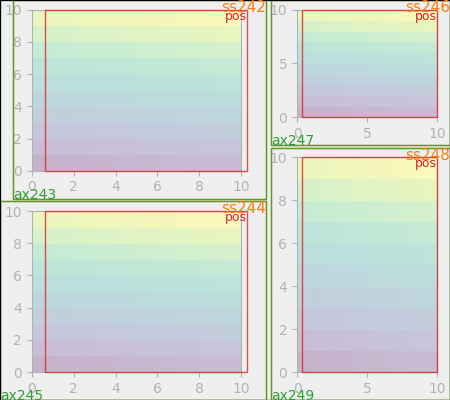### Empty gridspec slots¶

The final piece of the code that has not been explained is what happens if there is an empty gridspec opening. In that case a fake invisible axes is added and we proceed as before. The empty gridspec has no decorations, but the axes position in made the same size as the occupied Axes positions.

This is done at the start of `do_constrained_layout` (`hassubplotspec`).

```fig = plt.figure(constrained_layout=True)
gs = gridspec.GridSpec(1, 3, figure=fig)
im = ax.pcolormesh(arr, **pc_kwargs)